Courses

# Instruction Sets & Data Formats

## 10 Questions MCQ Test Topicwise Question Bank for Electronics Engineering | Instruction Sets & Data Formats

Description
This mock test of Instruction Sets & Data Formats for Electronics and Communication Engineering (ECE) helps you for every Electronics and Communication Engineering (ECE) entrance exam. This contains 10 Multiple Choice Questions for Electronics and Communication Engineering (ECE) Instruction Sets & Data Formats (mcq) to study with solutions a complete question bank. The solved questions answers in this Instruction Sets & Data Formats quiz give you a good mix of easy questions and tough questions. Electronics and Communication Engineering (ECE) students definitely take this Instruction Sets & Data Formats exercise for a better result in the exam. You can find other Instruction Sets & Data Formats extra questions, long questions & short questions for Electronics and Communication Engineering (ECE) on EduRev as well by searching above.
QUESTION: 1

### A microprocessor using a 3 MHz clock has three T ' states in each machine cycle. What is the time taken to complete the execution of an instruction if the instruction cycle of this instruction needs 4 machine cycles?

Solution:

Number of T-states in each machine cycle = 3 So, number of T-states in 4 machine cycles = 3 x 4 = 12

Given, f = 3 MHz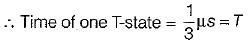Thus, time required for execution of the instruction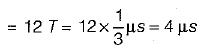QUESTION: 2

### Which of the following instruction will alter the contents of accumulator?

Solution:
• Instruction ‘CMP C’ compares the contents of register C with the content of accumulator A but contents of A and C will remain unchanged.
• Instruction ‘CPI 3A H' compares the 8-bit data 3A H with the contents of accumulator A but contents of A and C will remain unchanged.
• In instruction ‘AN! 5C H’, the 8-bit data 5C H is ANDed with contents of A and result is stored in A. So, the content of accumulator changes.
• In instruction ‘ORA A’, contents of A are ORed with contents of A itself and result is stored in A. But, the result of operation remains same as contents before execution ' of ORA A.

Hence, the contents of A does not change.

QUESTION: 3

### The addressing mode in which the machine language instruction itself includes the data is called

Solution:

In immediate addressing mode, an 8 bit or 16 bit data Is given In the instruction as operand.

QUESTION: 4

In an 8085 microprocessor, the accumulator contents are AAFI. After the execution of the instruction ‘CPI 99PT in the microprocessor

Solution:

Initially contents of accumulator A = AA H.
The instruction CPI 99 H compares the data 99H w ith the content of accumulator (A). This comparison is made by subtracting 99 H from contents of A i.e. AA H.
The contents of accumulator will remain unaffected but status of result will be reflected by flags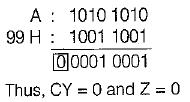Hence, after comparison both carry and zero flags will be reset

QUESTION: 5

The content of TEMP location after the execution of the following program in a 8085 microprocessor is:
MVI B, 00H
MVI A, IC H
DCR B
DAA
STA TEMP
HLT

Solution: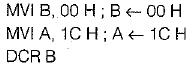Decrement the content of B by one (initially B = 00 H)

B= FF H

D A A ; D A A instruction changes the binary values of contents of accumulator to BCD.
Since lower order nibble of A is more than 9 (i.e. C), AC flag is set and upper nibble is less than 9, therefore DAA adds 0110 H to lower order nibble of A to adjust binary result to Binary coded decimal.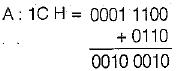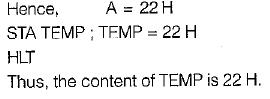QUESTION: 6

The following program is executed in the 8085 microprocessor: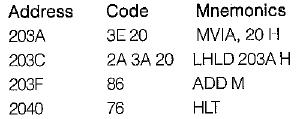What are the contents of the accumulator after the execution of above program?

Solution: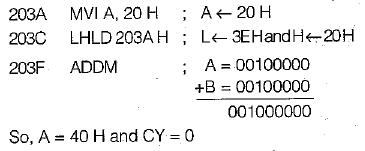2040 HLT ; Halts the execution of program Hence, the content of accumulator after the execution of given program are 40 H.

QUESTION: 7

What is the addressing mode used in the instruction PUSH D

Solution:

PUSH D stores the content of register pair DE on two top locations of stack, it is a 1 byte instruction and uses register indirect addressing mode.

QUESTION: 8

What is the effect of a DAD H instruction? ​

Solution:
QUESTION: 9

An instruction cycle is the time in which, a hardwired controlier completes four functions. The correct sequence of these functions is

Solution:

An instruction cycle is the time in which, a hardwired controller completes the following four functions;
(i) fetching of code from memory
(ii) updating the program counter
(iii) decoding of the instruction code
(iv) execution of the instruction

QUESTION: 10

Which of the following instruction does not use the stack?

Solution:

CALL and RET instruction uses stack while JMP instruction does not uses stack.